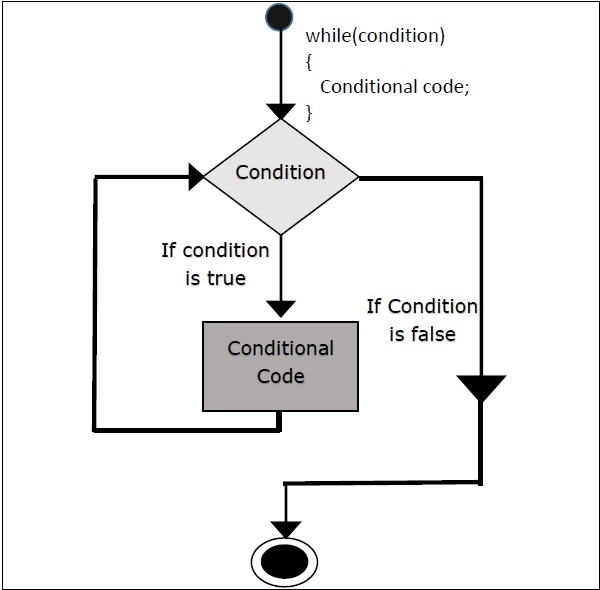# Scala - while Loop

Repeats a statement or group of statements while a given condition is true. It tests the condition before executing the loop body. A while loop statement repeatedly executes a target statement as long as a given condition is true.

## Syntax

The following is a syntax for while loop.

```while(condition){
statement(s);
}
```

Here, statement(s) may be a single statement or a block of statements. The condition may be any expression, and true is any nonzero value. The loop iterates while the condition is true. When the condition becomes false, program control passes to the line immediately following the loop.

## Flow ChartHere, key point of the while loop is that the loop might not ever run. When the condition is tested and the result is false, the loop body will be skipped and the first statement after the while loop will be executed.

Try the following example program to understand loop control statements (while statement) in Scala Programming Language.

## Example

```object Demo {
def main(args: Array[String]) {
// Local variable declaration:
var a = 10;

// while loop execution
while( a < 20 ){
println( "Value of a: " + a );
a = a + 1;
}
}
}
```

Save the above program in Demo.scala. The following commands are used to compile and execute this program.

## Command

```\>scalac Demo.scala
\>scala Demo
```

## Output

```value of a: 10
value of a: 11
value of a: 12
value of a: 13
value of a: 14
value of a: 15
value of a: 16
value of a: 17
value of a: 18
value of a: 19
```
scala_loop_types.htm